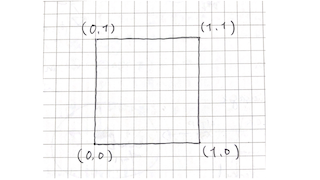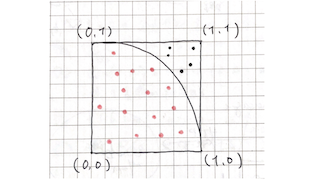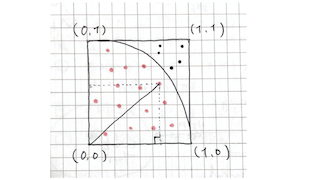# Finding pi with the Monte Carlo method? (Ruby)

The mentor advised me, "To learn a new language, try to find the pi using the Monte Carlo method!", So I decided to try it blindly.

# What are you saying? (Lol)

However, I didn't understand the meaning of the Japanese word "calculating the circumference ratio by the Monte Carlo method" at all, and I started by understanding it (laughs).

### Organize words

-** Monte Carlo method : Observe and analyze using random numbers. Monte Carlo seems to be the name of the district where the Principality of Monaco is located in Monte Carlo. - Random number : A number that is randomly output with equal probability within a fixed range of numbers. - Finding the circumference ratio : What I understood is to find the area of ​​the unit circle from the conclusion. - Unit circle **: A circle with a radius of 1

In summary, I understood that ** finding the circumference ratio by the Monte Carlo method ** means ** generating numbers randomly and analyzing them to find the area of ​​a circle with a radius of 1.

I still don't understand the meaning (laughs)

### How to find pi

So, here is a summary of the ideas of the smart people who were on the net.

(1) First, to find the area of ​​a circle with a radius of 1, divide the circle into four parts. (2) Then put it in a square with one side. (3) Take the square as coordinates and imagine it as shown in the figure below.(4) The bottom is a state with 1/4 yen. (Ignore the dots for a moment)(5) Randomly make dots in the upper square (see the dots). In other words, we will randomly type an array of (x, y). (6) Do this n times (many times). (7) Count the points (colored in pink) inside the circle and use it as p. (8) How to determine that a point is in a circle? Calculate the length of a random point `(x, y)` from the start point `(0, 0)`, and if this is 1 or less, judge it as inside a circle.(9) The length from `(0, 0)` to `(x, y)` can be calculated as the hypotenuse of a right triangle. Do you remember the three-square theorem? (I forgot) (10) After n times, divide p by n (p/n), which is an approximation of the area of ​​a 1/4 circle. (11) Multiply p/n by 4 to find the value of the circle.

# When you program

As for the code, I wrote it like this. (Please also refer to the advice of @scivola and @ kojix2 in the comments section)

#### `main.ruby`

``````
n = 1000000
count = 0

for i in 0 .. n
z = Math.sqrt((rand ** 2) + (rand ** 2))
if z < 1
count += 1
end
end

#Circumference
cir = count / n . to_f * 4 #to_The decimal point is not displayed unless it is set to float with f.

p cir
``````
• `Math` is a built-in module that groups mathematical methods.
• `.` Specify the receiver message (in this case, the message is`sqrt ()`)
• `Sqrt ()` is an abbreviation for square root. Similar to PHP.

# Afterword

I was 36 years old and changed jobs as an IoT engineer. Since that position is Ruby main, I put the familiar PHP and started studying Ruby.